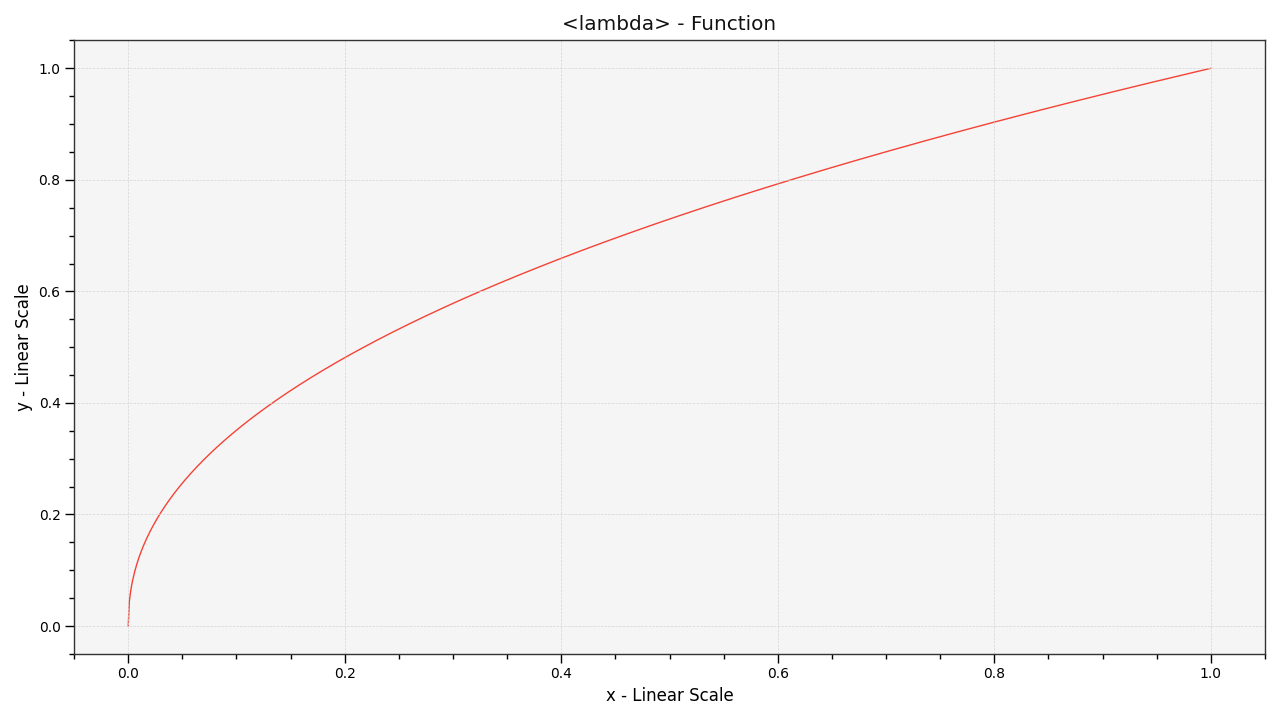# colour.plotting.plot_single_function#

colour.plotting.plot_single_function(function: Callable, samples: ArrayLike | None = None, log_x: = None, log_y: = None, plot_kwargs: dict | List[dict] | None = None, **kwargs: Any) Tuple[Figure, Axes][source]#

Plot given function.

Parameters:
Returns:

Current figure and axes.

Return type:

`tuple`

Examples

```>>> from colour.models import gamma_function
>>> plot_single_function(partial(gamma_function, exponent=1 / 2.2))
...
(<Figure size ... with 1 Axes>, <...Axes...>)
```